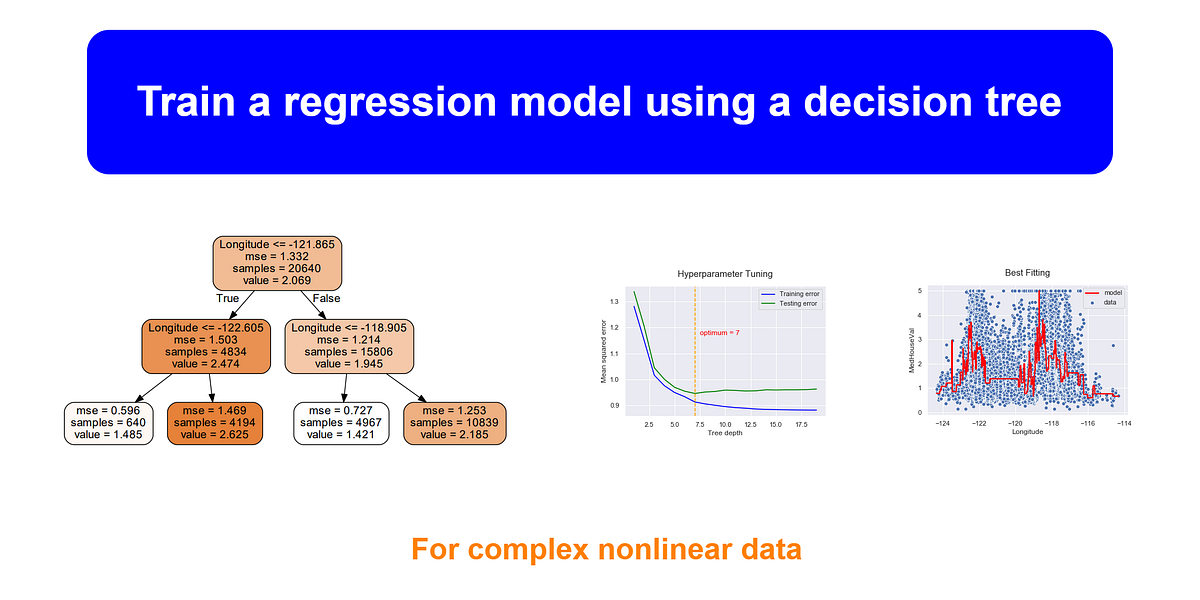# Train a regression model using a decision treewe only focus on decision trees with a regression task. For this, the equivalent Scikit-learn class is DecisionTreeRegressor. We will start by discussing how to train, visualize and make predictions with Decision Trees for a regression task. We will also discuss how to regularize hyperparameters in decision trees.

Decision Trees *are a non-parametric supervised learning method, capable of finding complex nonlinear relationships in the data. They can perform both classification and regression tasks. But in this article, we only focus on decision trees with a regression task. For this, the equivalent Scikit-learn class is *DecisionTreeRegressor.

We will start by discussing how to train, visualize and make predictions with Decision Trees for a regression task. We will also discuss how to regularize hyperparameters in decision trees. This will avoid the problem of overfitting. Finally, we will discuss some of the advantages and disadvantages of Decision Trees.

### Code convention

We use the following code convention to import the necessary libraries and set the plot style.

``````%matplotlib inline

import numpy as np
import pandas as pd
import seaborn as sns
import matplotlib.pyplot as plt

sns.set()``````

### Target audience

I assume that you have a basic understanding of the terminology used in the decision tree and how it works behind the scenes. In this tutorial, more emphasis is given for the model hyperparameter tuning techniques such as k-fold cross-validation.

## Getting Started with scikit-learn Pipelines for Machine Learning

Getting Started with scikit-learn Pipelines for Machine Learning: Building a pipeline from the ground up. (All code in this post is also included in this GitHub repository.)

## Most popular Data Science and Machine Learning courses — July 2020

Most popular Data Science and Machine Learning courses — August 2020. This list was last updated in August 2020 — and will be updated regularly so as to keep it relevant

## 15 Machine Learning and Data Science Project Ideas with Datasets

Learning is a new fun in the field of Machine Learning and Data Science. In this article, we’ll be discussing 15 machine learning and data science projects.

## Best Free Datasets for Data Science and Machine Learning Projects

This post will help you in finding different websites where you can easily get free Datasets to practice and develop projects in Data Science and Machine Learning.

## Visualize a Decision Tree in Machine Learning | Data Science | Machine Learning | Python

In the right side, we have a visualization of the output we get when we use a decision tree algorithm on data to predict the possibilities.